Updating search results...

# 81 Results

View
Selected filters:
• cac077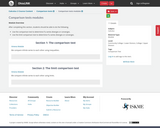Conditional Remix & Share Permitted
CC BY-NC
Rating
0.0 stars

After completing this section, students should be able to do the following.Use the comparison test to determine if a series diverges or converges.Use the limit comparison test to determine if a series diverges or converges.

Subject:
Calculus
Material Type:
Module
07/26/2019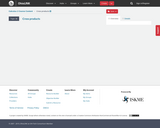Unrestricted Use
CC BY
Rating
0.0 stars
Rating
0.0 stars
Subject:
Calculus
Mathematics
Material Type:
Unit of Study
Provider:
Ohio Open Ed Collaborative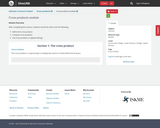Conditional Remix & Share Permitted
CC BY-NC
Rating
0.0 stars

After completing this section, students should be able to do the following.Define the cross product.Compute cross products.Use cross products in appled settings.

Subject:
Calculus
Material Type:
Module
07/26/2019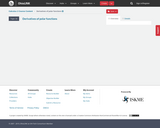Unrestricted Use
CC BY
Rating
0.0 stars
Rating
0.0 stars
Subject:
Calculus
Mathematics
Material Type:
Unit of Study
Provider:
Ohio Open Ed Collaborative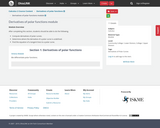Conditional Remix & Share Permitted
CC BY-NC
Rating
0.0 stars

After completing this section, students should be able to do the following.Compute derivatives of polar curves.Determine where the derivative of a polar curve is undefined.Find the equation of a tangent lines to a polar curve.

Subject:
Calculus
Material Type:
Module
07/26/2019Unrestricted Use
CC BY
Rating
0.0 stars
Rating
0.0 stars
Subject:
Calculus
Mathematics
Material Type:
Unit of Study
Provider:
Ohio Open Ed Collaborative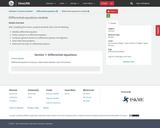Conditional Remix & Share Permitted
CC BY-NC
Rating
0.0 stars

After completing this section, students should be able to do the following.Identify a differential equation.Verify a solution to a differential equation.Compute a general solution to a differential equation via integration.Solve initial value problems.Determine the order of a differential equation.&nbsp;

Subject:
Calculus
Material Type:
Module
07/26/2019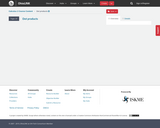Unrestricted Use
CC BY
Rating
0.0 stars
Rating
0.0 stars
Subject:
Calculus
Mathematics
Material Type:
Unit of Study
Provider:
Ohio Open Ed Collaborative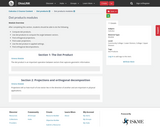Conditional Remix & Share Permitted
CC BY-NC
Rating
0.0 stars

After completing this section, students should be able to do the following.Compute dot products.Use dot products to compute the angle between vectors.Find orthogonal projections.Find scalar projections.Use the dot product in applied settings.Find orthogonal decompositions.

Subject:
Calculus
Material Type:
Module
07/26/2019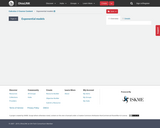Unrestricted Use
CC BY
Rating
0.0 stars
Rating
0.0 stars
Subject:
Calculus
Mathematics
Material Type:
Unit of Study
Provider:
Ohio Open Ed Collaborative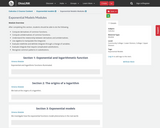Conditional Remix & Share Permitted
CC BY-NC
Rating
0.0 stars

After completing this section, students should be able to do the following.Compute derivatives of common functions.Compute antiderivatives of common functions.Understand the relationship between derivatives and antiderivatives.Use algebra to manipulate the integrand.Evaluate indefinite and definite integrals through a change of variables.Evaluate integrals that require complicated substitutions.Recognize common patterns in substitutions.

Subject:
Calculus
Material Type:
Module
07/25/2019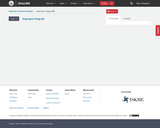Unrestricted Use
CC BY
Rating
0.0 stars
Rating
0.0 stars
Subject:
Calculus
Mathematics
Material Type:
Unit of Study
Provider:
Ohio Open Ed Collaborative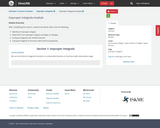Conditional Remix & Share Permitted
CC BY-NC
Rating
0.0 stars

After completing this section, students should be able to do the following.Identify an improper integral.Determine if an improper integral converges or diverges.Compute integrals over infinite intervals.Compute integrals of functions with vertical asymptotes.

Subject:
Calculus
Material Type:
Module
07/25/2019Unrestricted Use
CC BY
Rating
0.0 stars
Rating
0.0 stars
Subject:
Calculus
Mathematics
Material Type:
Unit of Study
Provider:
Ohio Open Ed Collaborative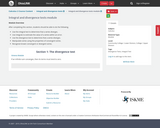Conditional Remix & Share Permitted
CC BY-NC
Rating
0.0 stars

After completing this section, students should be able to do the following.Use the integral test to determine that a series diverges.Use integrals to estimate the value of a series within an error.Use the divergence test to determine that a series diverges.Manipulate series using the properties of convergent series.Recognize known convergent or divergent series.

Subject:
Calculus
Material Type:
Module
07/26/2019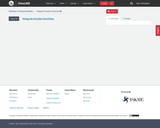Unrestricted Use
CC BY
Rating
0.0 stars
Rating
0.0 stars
Subject:
Calculus
Mathematics
Material Type:
Unit of Study
Provider:
Ohio Open Ed Collaborative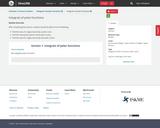Conditional Remix & Share Permitted
CC BY-NC
Rating
0.0 stars

After completing this section, students should be able to do the following.Find the area of a region bound by a polar curve.Find the intersection points of two polar curves.Find the area of a region bound by two polar curves.

Subject:
Calculus
Material Type:
Module
07/26/2019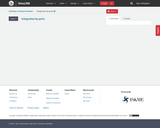Unrestricted Use
CC BY
Rating
0.0 stars
Rating
0.0 stars
Subject:
Calculus
Mathematics
Material Type:
Unit of Study
Provider:
Ohio Open Ed CollaborativeConditional Remix & Share Permitted
CC BY-NC
Rating
0.0 stars

After completing this section, students should be able to do the following.Describe the relationship between the product rule and integration by parts.Identify the functions required to transform the integral via integration by parts.Evaluate integrals using integration by parts, including multiple iterations.Use integration by parts to derive reduction formulas.

Subject:
Calculus
Material Type:
Module
07/25/2019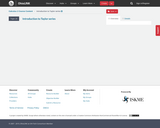Unrestricted Use
CC BY
Rating
0.0 stars
Rating
0.0 stars
Subject:
Calculus
Mathematics
Material Type:
Unit of Study
Provider:
Ohio Open Ed Collaborative